# Thread: complicated logarithmic differentiation

1. ## complicated logarithmic differentiation

i'm so sorry for keep asking questions but im really confused for this one.
the problem is y=(e^(x-1)*sin^2*x)/(((x^2)+5)^2x)

the trig function (sin^2 + 5), i don't know how to get a derivative out of that.

help me plz!

2. Hi katieeej -

if you mean $\displaystyle \sin^2 x + 5$ then this calls for a simple application of the chain rule for differentiation...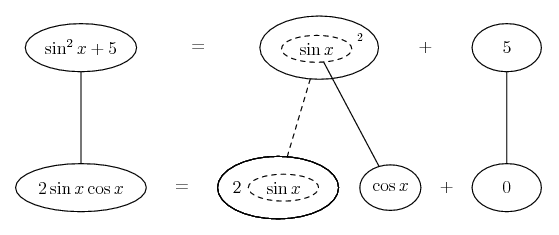...where straight continuous line differentiate downwards with respect to x and the straight dashed line with respect to the dashed balloon expression, so that the triangular network satisfies the chain rule...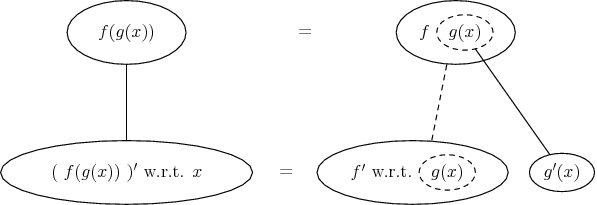... though I'm not sure you meant \sin^2 x...

Don't integrate - balloontegrate!

Balloon Calculus: worked examples from past papers

3. ## clarification

the equation is

y=

e^(x-1)*sin^2(x)
-------------------- division
(x^2 +5)^2x

i started off like
ln y = ln(e^(x-1)sin^2 (x)) - ln (x^2 +5)^2x
= ln(e^(x-1))+ln (sin^2 (x))- 2x ln (x^2 +5)
= x-1 ln e +ln sin^2 (x) - 2x ln (x^2+5)
=x-1+ln (sin^2 (x)) -2x ln (x^2 +5)

then i don't know how to get derivatives.

4.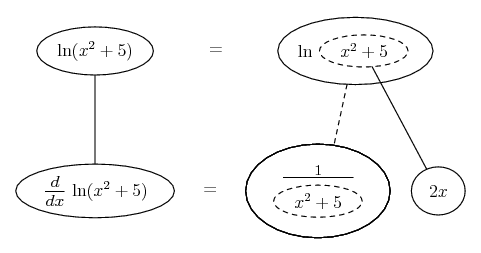More in a moment...

5. Then the product rule...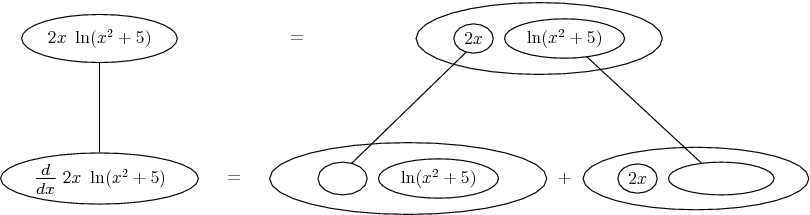And then you need to apply the chain rule to $\displaystyle \ln | \sin^2 x |$...

6. And using the derivative of $\displaystyle \sin^2 x$ which is the same as that of $\displaystyle \sin^2 x + 5$ in the first picture...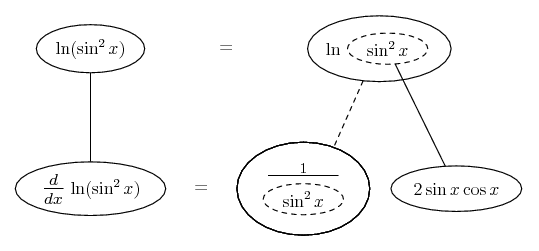Don't integrate - balloontegrate!

Balloon Calculus: worked examples from past papers

#### Search Tags

complicated, differentiation, logarithmic